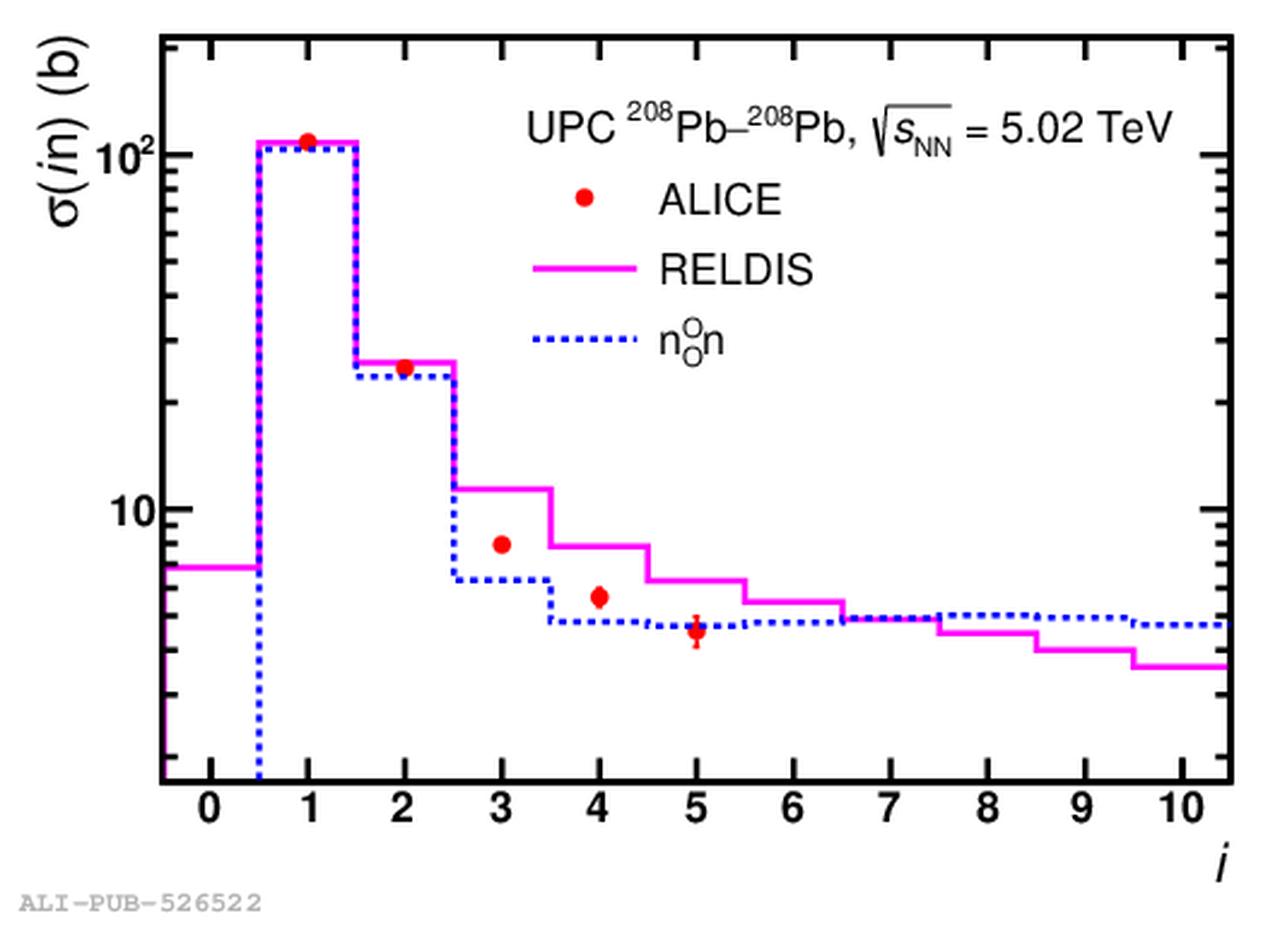# Figure 2

 Colour online: measured (points) and calculated with RELDIS  (solid-line histogram) and $\mathrm{n^O_On}$  (dashed-line histogram) cross sections of emission of given numbers of neutrons $i$ in UPCs of $^{208}$Pb nuclei at $\sqrt{s_{\mathrm{NN}}}=5.02$ TeV. Combined statistical and systematic uncertainties of the measurements are presented..